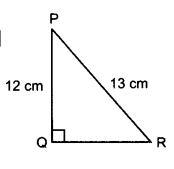" ">

# In given figure, find $tan\ P - cot\ R$."

Given:

$PQ = 12\ cm, PR = 13\ cm$.

To do:

We have to find $tan\ P - cot\ R$.

Solution:

We know that,

In a right-angled triangle $PQR$ with a right angle at $B$,

By Pythagoras theorem,

$PR^2=PQ^2+QR^2$

By trigonometric ratios definitions,

$tan\ P=\frac{Opposite}{Adjacent}=\frac{QR}{PQ}$

$cot\ R=\frac{Adjacent}{Opposite}=\frac{QR}{PQ}$

Here,

$PR^2=PQ^2+QR^2$

$\Rightarrow (13)^2=(12)^2+QR^2$

$\Rightarrow QR^2=169-144$

$\Rightarrow QR=\sqrt{25}=5$

Therefore,

$tan\ P=\frac{QR}{PQ}=\frac{5}{12}$

$cot\ R=\frac{QR}{PQ}=\frac{5}{12}$

Therefore,

$tan\ P - cot\ R = \frac{5}{12} - \frac{5}{12}$

$=0$

Updated on: 10-Oct-2022

13 Views